# PID-Based Enhanced Flower Pollination Algorithm Controller for Drilling Process in a Composite Material

PID-Based Enhanced Flower Pollination Algorithm Controller for Drilling Process in a Composite Material
Control and Systems Department, University of Technology-Iraq, Baghdad 10066, Iraq

Corresponding Author Email:
Page:
91-96
|
DOI:
https://doi.org/10.18280/acsm.470205
27 February 2023
|
Revised:
3 April 2023
|
Accepted:
8 April 2023
|
Available online:
30 April 2023
| Citation

OPEN ACCESS

Abstract:

Due to the variability in the physical and chemical properties of the composite material, understanding the dynamics of the drilling process in this material can be challenging. One of the most significant issues that can result from size and shape abnormalities in the hole during the drilling process is delamination. These errors could be unacceptable and lower the product's quality. In order to regulate the drilling process of Glass Fiber Reinforced Plastic (GFRP) composite, this work proposes an optimal Proportional-Integral-Derivative (PID) controller based on Enhanced Flower Pollination Algorithm (EFPA). Based on the Integral Time of Absolute Error (ITAE) index, the proposed tuning approach is compared with the traditional Flower Pollination Algorithm (FPA) and Particle Swarm Optimization (PSO). In terms of time response specifications and error performance index. Simulation results using MATLAB demonstrate the superiority of the proposed EFPA over conventional FPA and PSO for improving the tuning of the PID for controlling the drilling process.

Keywords:

drilling process, composite material, delamination, enhanced flower pollination algorithm

1. Introduction

Drilling is thought of as the secondary machining process for fiber-reinforced materials that is utilized the most frequently due to the need for linking pieces . Due to the differences in the physical and chemical characteristics of the constituents, understanding the mechanism of material removal is rather difficult. For a very long time, humans have used materials including glasses, polymers, ceramics, and metal alloys. There are no qualities like high specific strength or high stiffness in these typical materials. The requirement for materials that exhibit such a broad range of traits as a result of technological breakthroughs led to the creation of composite materials, which offer an unusual combination of qualities . Composite materials offer a number of advantages, including high strength-to-weight ratios, stiffness-to-weight ratios, exceptional chemical and corrosion resistance, superior impact characteristics, excellent mechanical qualities, and, most importantly, greater design flexibility. As a result of its electrical insulating properties, glass fiber reinforced plastic is frequently utilized in appliances, printed wiring boards, machine tool components, etc. .

As opposed to drilling metals, drilling composite materials requires the drill to alternatively move through fiber (reinforcement) and plastic (matrix), which have different properties. During the drilling of the composite materials, a series of fractures is produced that is assisted by the uneven and varying matrix and fiber force distribution . This phenomena of fractures in the composite materials during drilling is namned delamination and it is considered one of the main problems in the drilling. Composite materials delaminate at the entrance and exit of drilled holes . A range of measures has been established by various studies to assess this delamination damage. Among them are the damage ratio, minimal delamination factor, similar delamination factor, adjusted delamination factor, refined delamination factor, and so forth. The delamination factor seems simple and capable of explaining the distinctive delamination characteristics among all of these other assessment variables .

Thrust force must be controlled since it plays a significant role in delamination. The thrust force generated during the drilling process affects the delamination zone's size . Below a certain "critical thrust force," no harm is done. The feed rate affects the thrust force that is given to the composite laminate during drilling. As a result, the feed rate has a significant impact on the sort of damage that is caused to the composite material during drilling. In order to avoid damage and produce a hole of good quality, the feed rate must be carefully chosen because it is the main element to produce thrust force .

In order to model the dynamics of drilling composite materials, numerous strategies have been adopted. For instance, Stone and Krishnamurthy  suggested using neural networks to model the drilling procedure for the laminates made of graphite and epoxy. The thrust force responses for a variety of quasi-isotropic woven carbon fiber/epoxy laminates carbide-tipped twist drills were obtained by Dharan and Won  based on experimental data. Fuzzy logic was utilized by Chung and Tomizuka  to model the drilling of the composite material. Singh and Sharma  established a first-order system for glass fiber-reinforced plastic composite based on system identification. Later, Singh et al.  created a third-order state-space model for glass fiber-reinforced plastic composite.

Numerous control systems, including neural network controllers , intelligent control strategies , fuzzy logic controllers (FLC) , proportional-integral-derivative (PID) controllers , and optimum control , have been developed to prevent delamination. The traditional PID controller has a straightforward design and can enhance the system's response. However, the proportional, integral, and derivative gains, among other control parameters, are largely responsible for the PID controllers' performance . To identify the best value for the adjusted gains in the PID controller, a number of tuning techniques based on swam-based optimization have been presented in this direction. The drilling process of a glass fiber-reinforced plastic composite is controlled by a PID controller suggested in this research that is tuned by a brand-new swarm optimization called an Enhanced Flower Pollination Algorithm (EFPA). Based on time response parameters and an error performance index, the suggested tuning method is contrasted with the traditional Flower Pollination Algorithm (EPA) and Particle Swarm Optimization (PSO). The simulation is run with MATLAB software.

2. System Modeling

Drilling tests are performed using a radial drilling machine. The experimental set-up consists of the drilling machine, holding fixture, piezoelectric drill dynamometer (of the Kistler® brand), charge amplifier, connecting cables, analog to digital (A/D) converter, and computer. Utilizing a four-part drill dynamometer (Model: Kistler 9272®), the thrust force signals are recorded. These signals are then enhanced using a charge amplifier (Model: Kistler 5070®) . The signal is then transmitted from the amplifier to the computer system using signal conditioning tools and a data capture card. Through the use of specialist data collection and analysis software (Dyno-Ware®), thrust force signals are recorded and examined. A table made of a 12 mm thick of GFRP composite laminate is being drilled with an 8 mm diameter tungsten carbide drill as it is being held in a rigid fixture connected to a dynamometer installed on a machine. In order to firmly mount a dynamometer on a machine table, square-headed bolts are inserted into T-slots .

To simulate drilling of GFRP composites, a SISO system has been created. It is possible to obtain first-order dynamics that represent the system based on knowledge of the drilling operation. A feed rate has been used as an input in the calculation of the thrust forces produced during drilling. A step shift in the response is commonly employed to characterize a system's dynamics for simplicity and robustness . This work employs the drilling process mathematical model that was created in the study . Figure 1 demonstrates how to drill glass fiber-reinforced plastic laminates that are 12 mm thick using a 212.8 mm/min step feed input. The system's first-order dynamics are predicted using transfer functions between the thrust force and feed rate using MATLAB system identification tools. Based on the thrust force response, the study  provides the first-order transfer function between thrust force and feed rate:

$\mathrm{G}(\mathrm{s})=\frac{\mathrm{F}(\mathrm{s})}{\mathrm{u}(\mathrm{s})}=\frac{1.1884}{0.4172 \mathrm{~s}+1}$                 (1)

where,

s      Laplace operator

G(s) Drilling-process transfer function

F      Cutting force

u      Feed rate

## 1.png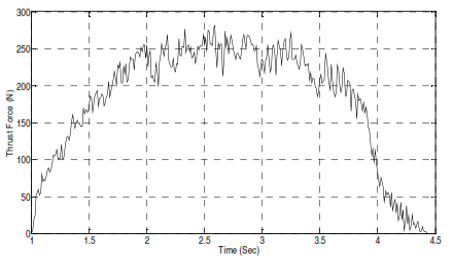Figure 1. Experimental thrust force response at feed rate of 212.8 mm/min 

3. Controller Design

Industrial control systems frequently use PID controllers, a sort of control loop feedback mechanism. The difference between the measured process variable and the desired set point is used to determine the error value . Proportional, integral, and derivative values are the three different parameters needed for the PID controller computation. The response to the present error is determined by the balanced value, the total of recent errors is used by the excellent information deal to establish the response, and the rate of change of the error is used by the derivative value to decide the response. Through the last control element, the process is modified using the weighted sum of these three actions . Figure 2 shows the general PID controller block diagram . The error (e (t)), which is the difference between the set-point and process output, and the control law (u (t)), are the input and output of the controller respectively.

## 2.pngFigure 2. The block diagram of PID controller

The goal of PID controller tuning is to find settings that meet the performance requirements for closed-loop systems while also maintaining the control loop's dependable operation under a variety of operating conditions. In reality, it is frequently challenging to simultaneously have all of these perfect qualities. A delayed response is produced when disturbed, for instance, when the PID controller is modified to provide a better transient reaction to set point change often. According to the study , the PID controller has the following standard form:

$u(t)=K_p e(t)+K_i \int_0^t e(t) d t+K_d \frac{d e}{d t}$                    (2)

where,

u(t)    Control Law

e(t)    Error

Kp      Proportional gain

Ki       Integral gain

Kd      Derivative gain

4. Meta-Heuristic Optimizations

Today, PID schemes offer the most straightforward and efficient answers to the majority of control engineering problems. PID controllers are, however, typically poorly tuned because it takes a lot of time and effort to adjust them manually. The turning process of the PID controller is formulated as an optimization problem using the most recent swam-based optimization technique. Figure 3 provides the block diagram for the intelligent PID controller that proposed in this work. In addition to the PSO, the FPA, and the new planned EPFA is explained in this section. In this work, these algorithms are utilized to determine the best value for the PID controller's adjusted gains.

## 3.png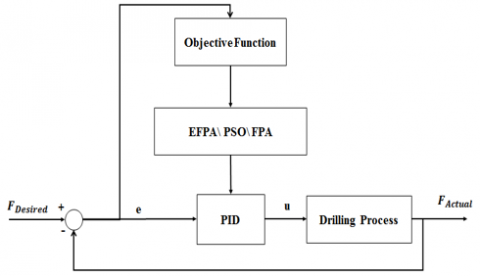Figure 3. Block diagram of the Intelligent PID controller

4.1 Particle Swarm Optimization (PSO)

PSO is an evolutionary computing-based optimization strategy. Swarm research, which includes investigations of fish schooling and avian swarming, forms the basis of the primary PSO . A modified PSO was produced in 1995 to enhance the functionality of the original PSO after its initial release in 1995 . Shi and Eberhart  introduced the inertia weight parameter. In the PSO, the algorithm searches the search space with a swarm of particles to get the best answer. Every particle in the search space modifies its "flying" according to its own flying experiences as well as those of other particles. The initial value for each particle's position and velocity in the PSO algorithm is a random number. The algorithm then updates the position and speed of each particle in each generation and seeks optimum values. Based on the position of the global best solution and local best solution, each particle's velocity is changed as follows :

$\mathrm{v}_{\mathrm{i}}(\mathrm{k}+1)=\theta \mathrm{v}_{\mathrm{i}}(\mathrm{k})+\mathrm{c}_1 \mathrm{r}_1\left(\mathrm{p}_{\mathrm{best}, \mathrm{i}}-\mathrm{x}_{\mathrm{i}}(\mathrm{k})\right)+\mathrm{c}_2 \mathrm{r}_2\left(\mathrm{~g}_{\mathrm{best}}-\mathrm{x}_{\mathrm{i}}(\mathrm{k})\right)$             (3)

where,

k         Index for iteration

i          Index for particle

v         Velocity of the particle

θ         Inertia weight coefficient decrease linearly from 0.9 to 0.4

c1,c2   Cognitive and social coefficients

r1,r2    Random value between [0, 1]

x          Position of the particle

pbest   Best position found by the individual particle

gbest    Best position found by the population

Based on the updated velocity, the new position of each particle is updated by:

$x_i(\mathrm{k}+1)=x_i(\mathrm{k})+v_i(\mathrm{k}+1)$                   (4)

4.2 Flower Pollination Algorithm (FPA)

The Flower Pollination Algorithm (FPA), a population-based approach motivated by nature, was suggested by Yang . By ensuring that the best blossoms among flower plants survive, flower pollination serves the fundamental function of ensuring that plants reproduce as successfully as possible. The primary function of a flower is to reproduce through pollination. Flower pollination is frequently linked to the transport of pollen, and pollinators including insects, birds, bats, and other animals are important in this process. The two basic types of pollination are abiotic and biotic. Nearly 90% of flowering plants use biological pollination, which implies that pollen is delivered by pollinators like insects and animals. Approximately 10% of all pollination is abiotic, or without the use of pollinators. Self-pollination and cross-pollination are the two processes that cause pollination. When pollen from a flower on a different plant is utilized to fertilize a single blossom, this process is known as cross-pollination. In contrast, self-pollination takes place when a single flower is fed by pollen from another flower on the same plant or from the same flower itself . The algorithm begins by placing a population of N flowers in a random location inside the search space. The method makes use of two probability-based search algorithms. Making use of a round value (Rand). The FPA conducts a global search if >Rand. Each individual agent in the population has its position in the population's pollination search altered by the position of the best answer as shown below :

$\mathrm{p}_{\mathrm{i}}(\mathrm{k}+1)=\mathrm{p}_{\mathrm{i}}(\mathrm{k})+\sigma\left(\mathrm{p}_{\mathrm{g}}-\mathrm{p}_{\mathrm{i}}(\mathrm{k})\right)$                   (5)

where,

p      Position of individual solution

σ      Step size

pg     Best position found by the population

If $\beta \leq Rand$, the FPA performs a local search. In the local pollination search, the each individual agent in the population is adjusted its position by selecting two solutions randomly from the population as follows :

$\mathrm{p}_{\mathrm{i}}(\mathrm{k}+1)=\mathrm{p}_{\mathrm{i}}(\mathrm{k})+\epsilon\left(\mathrm{p}_{\mathrm{i}}-\mathrm{p}_{\mathrm{q}}\right)$               (6)

where,

ϵ         Random value between [0,1]

pj, pq  Position of two solutions selected randomly from the population

4.3 Enhanced Flower Pollination Algorithm (EFPA)

An improved version of the FPA is presented in this research in order to increase the algorithm's search process. Each agent in the EFPA altered its position depending on the optimal position that has been discovered by the algorithm, as opposed to using the agent's existing position to find the new position, as in the original FPA for local and global search. As a result, Eq. (5) is changed to Eq. (7) for the global search. Similarly, Eq. (6) is changed to Eq. (8) for the local search.

$\mathrm{p}_{\mathrm{i}}(\mathrm{k}+1)=\mathrm{p}_{\mathrm{g}}+\sigma\left(\mathrm{p}_{\mathrm{i}}(\mathrm{k})-\mathrm{p}_{\mathrm{g}}\right)$                (7)

$\mathrm{p}_{\mathrm{i}}(\mathrm{k}+1)=\mathrm{p}_{\mathrm{g}}+\epsilon\left(\mathrm{p}_{\mathrm{i}}(\mathrm{k})-\mathrm{p}_{\mathrm{j}}\right)$                  (8)

This enhancement improves the convergence of the algorithm towards the optimal solution.

5. Simulation Results

The simulation results of controlling the drilling process's cutting force for GFRP are provided in this section. The transfer function of the GFRP drilling process found in Eq. (1) is utilized to carry out the simulation. The process is controlled by the PID controller specified in Eq. (2). An optimization problem is used to formulate the process of tuning the PID controller. In addition to the PSO, FPA, and the suggested EPFA are employed to determine the PID parameters' gain values. The Integral Time of Absolute Error (ITAE) index, which is given in Eq. (9) [20, 21] is used as the cost function in the optimization to enhance the tuning process.

ITAE $=\int_{t=0}^{\mathrm{t}=\mathrm{t}_{\text {sim }}} \mathrm{t}|\mathrm{e}(\mathrm{t})| \mathrm{dt}$                   (9)

where, tsim  is the simulation time and e(t) refers to the error in the drilling process measured as the deviation of the actual cutting force from the desired cutting force. The parameters of the EPFA, PSO and FPA are listed in Table 1.

Table 1. Algorithm parameters of PSO, EPFA, and FPA

 Parameters Values PSO FPA EFPA Population Size (N) 25 25 25 Number of Iterations (Tmax) 40 40 40 Probability ($\beta$) - 0.5 0.5 Inertia Weight (wmax) 0.9 - - Inertia Weight (wmin) 0.4 - - Cognitive Learning Rate (c) 2 - - Step Size ($\sigma$) - 2 2

Table 2. PID parameters values using PSO, FPA and EFPA

 Tuning Method PID Parameters Kp Ki Kd PSO 27.8 54.4 2.14 FPA 27.57 38.61 2.74 EFPA 28.87 60 1.6

The three controller structures are subjected to a unit step change in the required input force in order to assess the suggested EFPA algorithm to tune the PID controller for the drilling process of the composite material. Table 2 lists the values for the PID controller's gains Kp, Ki, and Kd based on the three tuning procedures. Figure 4 shows the response of the PID-PSO, PID-FPA, and PID-EFPA controllers to an input of unit step. Additionally, Table 3 displays the system's performance index for the unit step based on the time domain and ITAE.

## 4.png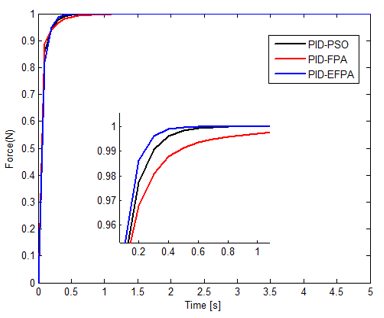Figure 4. Unit step response of PID-PSO, PID-FPA and PID-EFPA

Table 3. Performance index of the system for unit step

 Tuning Method Performance Index ts Mp ITAE PSO 0.5 0 0.4 FPA 2.2 0 0.965 EFPA 0.35 0 0.344

There is no overshoot for the three responses of the system, as shown in Table 3 and Figure 4. However, EFPA-PID settles more quickly than FPA-PID and PSO-PID. Additionally, the EFPA-ITAE PID's is lower than that of the FPA-PID and PSO-PID. These findings show that EFPA delivers greater performance when tuning PID for GFRP drilling than FPA and PSO. In the second instance, it was assumed that there existed a critical force, after which the drilling process would experience a delamination issue. As a result, the system's reference force input should be set lower than this critical force. As a result, Figure 5 depicts the reference force input for drilling the GFRP and the critical force profile.

## 5.png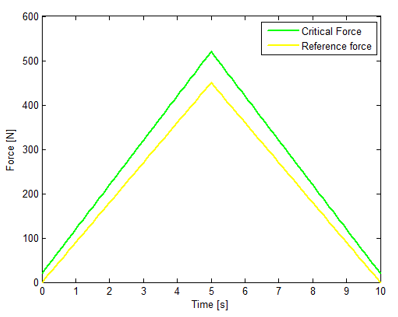Figure 5. Critical and reference force for drilling process

Table 2 lists the prior values of the intended gains $\mathrm{K}_{\mathrm{p}} \mathrm{K}_{\mathrm{i}}$ and $\mathrm{K}_{\mathrm{d}}$ of the PID controller that were achieved in the second case using unit step input. The response of the PID-PSO, PID-FPA, and PID-EFPA controllers to the reference force input is shown in Figure 6. Additionally, Table 4 provides the ITAE performance index.

Based on Table 4, it can be seen that the ITAE of the EFPA-PID is less than FPA-PID and PSO-PID. This proves that tuning PID for drilling GFRP by EFPA achieves better performance that FPA and PSO.

## 6.png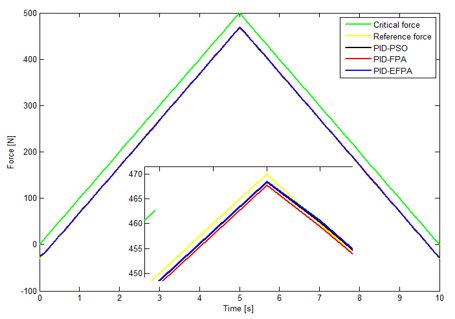Figure 6. System response of PID-PSO, PID-FPA and PID-EFPA for reference force input

Table 4. Performance index of the system for reference force input

 Tuning Method ITAE PSO 154.52 FPA 201.39 EFPA 142.92
6. Conclusions

In order to regulate the thrust force of the drilling process of composite materials, a control strategy based on a PID controller tuned by a new swarm optimization named Enhanced Flower Pollination Algorithm (EFPA) is proposed in this study. The controller's goal is to keep drilling from experiencing delamination issues. The suggested tuning method is compared with tuning achieved from the traditional EPA and PSO based on the ITAE index when the required input force is a unit step and follows a reference force profile in order to evaluate the proposed algorithm. The comparison of the final results between the EPA, PSO, and newly proposed EFPA algorithms as tuning to the PID controller parameters shows that the PID tuned by EFPA controller results are more striking than those of the others. The EFPA-ITAE PID's index value is lower for the unit step and the reference force profile when compared to the measured values for the PSO-PID and FPA-PID. These results demonstrate the effectiveness of the newly suggested optimization technique to adjust the PID for drilling composite materials.

References

 Kyratsis, P., Markopoulos, A.P., Efkolidis, N., Maliagkas, V., Kakoulis, K. (2018). Prediction of thrust force and cutting torque in drilling based on the response surface methodology. Machines, 6(2): 24. https://doi.org/10.3390/machines6020024

 Singh, A.P., Sharma, M., Singh, I. (2013). A review of modeling and control during drilling of fiber reinforced plastic composites. Composites Part B: Engineering, 47: 118-125. https://doi.org/10.1016/j.compositesb.2012.10.038

 Malik, K., Ahmad, F., Keong, W.T., Gunister, E. (2022). The effects of drilling parameters on thrust force, temperature and hole quality of glass fiber reinforced polymer composites. Polymers and Polymer Composites, 30: 09673911221131113. https://doi.org/10.1177/09673911221131113

 Singh, A.P., Sharma, M. (2014). Modeling and PID control of thrust force during drilling in composite laminates. In 2014 Recent advances in engineering and computational sciences (RAECS), pp. 1-5. Chandigarh, India. https://doi.org/10.1109/RAECS.2014.6799513

 Kilickap, E. (2010). Optimization of cutting parameters on delamination based on Taguchi method during drilling of GFRP composite. Expert systems with applications, 37(8): 6116-6122. https://doi.org/10.1016/j.eswa.2010.02.023

 Babu, J., Alex, N.P., Mohan, K.P., Philip, J., Davim, J.P. (2016). Examination and modification of equivalent delamination factor for assessment of high speed drilling. Journal of Mechanical Science and Technology, 30: 5159-5165. https://doi.org/10.1007/s12206-016-1034-4

 Stone, R., Krishnamurthy, K. (1996). A neural network thrust force controller to minimize delamination during drilling of graphite-epoxy laminates. International Journal of Machine Tools and Manufacture, 36(9): 985-1003. https://doi.org/10.1016/0890-6955(96)00013-2

 Dharan, C.K.H., Won, M.S. (2000). Machining parameters for an intelligent machining system for composite laminates. International Journal of Machine Tools and Manufacture, 40(3): 415-426. https://doi.org/10.1016/S0890-6955(99)00065-6

 Chung, B.M., Tomizuka, M. (2001). Fuzzy logic modeling and control for drilling of composite laminates. In 10th IEEE International Conference on Fuzzy Systems.(Cat. No. 01CH37297), 1: 509-512. Melbourne, VIC, Australia. https://doi.org/10.1109/FUZZ.2001.1007360

 Singh, A.P., Sharma, M., Singh, I. (2017). Optimal control of thrust force for delamination-free drilling in glass-fiber-reinforced plastic laminates. Proceedings of the Institution of Mechanical Engineers, Part B: Journal of Engineering Manufacture, 231(13): 2396-2407. https://doi.org/10.1177/0954405416629864

 Ahamed, S.R., Parumasivam, P., Lipu, M.H., Hannan, M.A., Ker, P.J. (2020). A comparative evaluation of PID-based optimisation controller algorithms for DC motor. International Journal of Automation and Control, 14(5-6): 634-655.

 Ahmed, S., Huang, B., Shah, S.L. (2007). Novel identification method from step response. Control Engineering Practice, 15(5): 545-556. https://doi.org/10.1016/j.conengprac.2006.10.005

 Nagaraj, B., Murugananth, N. (2010). A comparative study of PID controller tuning using GA, EP, PSO and ACO. In 2010 International Conference On Communication Control And Computing Technologies, pp. 305-313. Nagercoil, India. https://doi.org/10.1109/ICCCCT.2010.5670571

 Al-Khazraji, H., Cole, C., Guo, W. (2018). Multi-objective particle swarm optimisation approach for production-inventory control systems. Journal of Modelling in Management, 13(4): 1037-1056. https://doi.org/10.1108/JM2-02-2018-0027

 Eberhart, R., Kennedy, J. (1995). A new optimizer using particle swarm theory. In MHS'95. Proceedings of the Sixth International Symposium on Micro Machine and Human Science, pp. 39-43. Nagoya, Japan. https://doi.org/10.1109/MHS.1995.494215

 Shi, Y., Eberhart, R. (1998). A modified particle swarm optimizer. In 1998 IEEE international conference on evolutionary computation proceedings. IEEE world congress on computational intelligence (Cat. No. 98TH8360), pp. 69-73. Anchorage, AK, USA. https://doi.org/10.1109/ICEC.1998.699146

 Yang, X.S. (2012). Flower pollination algorithm for global optimization. In Unconventional Computation and Natural Computation: 11th International Conference, UCNC 2012, Orléan, France, pp. 240-249. https://doi.org/10.1007/978-3-642-32894-7_27

 Alyasseri, Z.A.A., Khader, A.T., Al-Betar, M.A., Awadallah, M.A., Yang, X.S. (2018). Variants of the flower pollination algorithm: a review. Nature-Inspired Algorithms and Applied Optimization, 744: 91-118. https://doi.org/10.1007/978-3-319-67669-2_5

 Al-Khazraji, H. (2022). Comparative study of whale optimization algorithm and flower pollination algorithm to solve workers assignment problem. International Journal of Production Management and Engineering, 10(1): 91-98. https://doi.org/10.4995/ijpme.2022.16736

 Al-Khazraji, H. (2022). Optimal design of a proportional-derivative state feedback controller based on meta-heuristic optimization for a quarter car suspension system. Mathematical Modelling of Engineering Problems, 9(2): 437-442. https://doi.org/10.18280/mmep.090219

 Abood, L.H., Oleiwi, B.K. (2021). Design of fractional order PID controller for AVR system using whale optimization algorithm. Indonesian Journal of Electrical Engineering and Computer Science, 23(3): 1410-1418. https://doi.org/10.11591/ijeecs.v23.i3.pp1410-1418